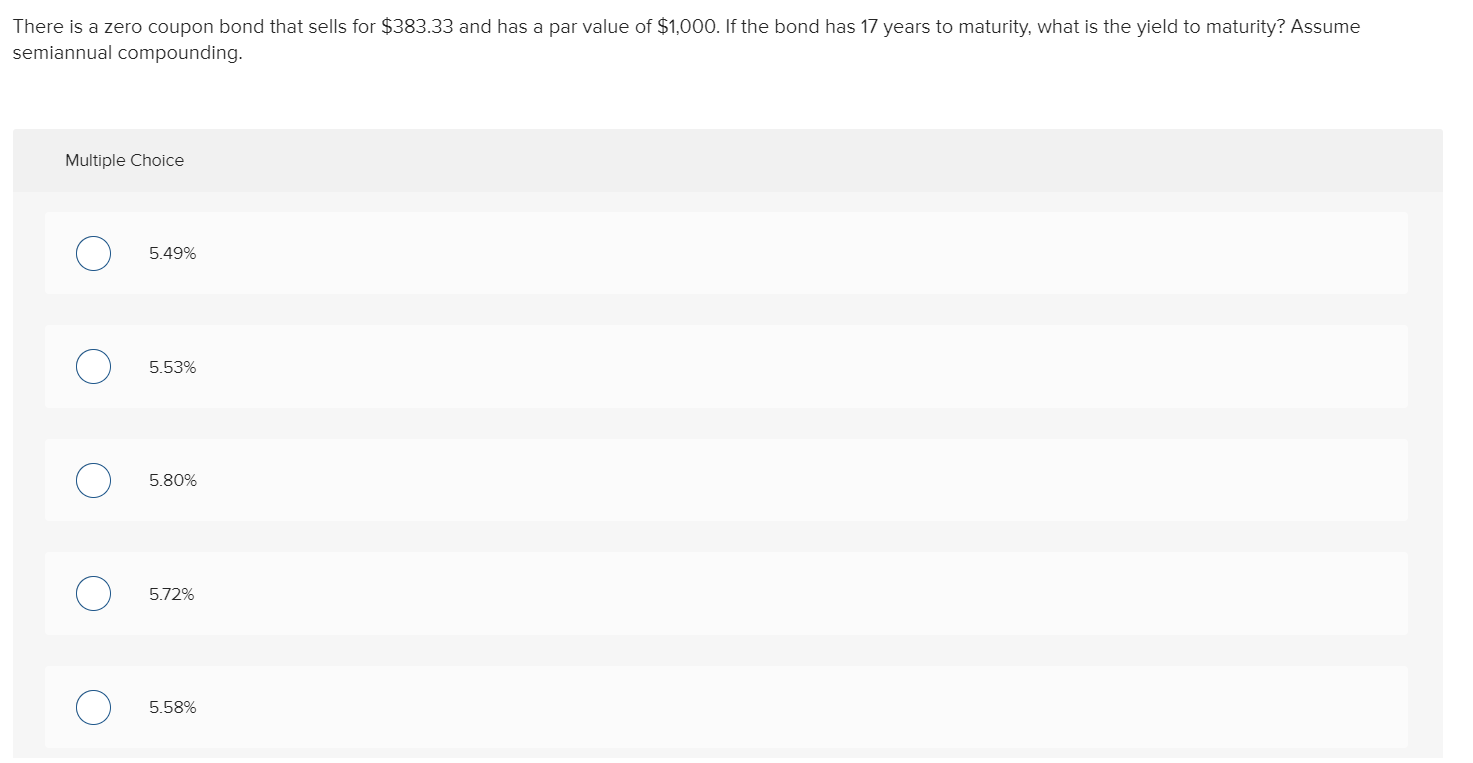Question

What is the yield on a zero-coupon bond with a par value of \$1,000, a price...

What is the yield on a zero-coupon bond with a par value of \$1,000, a price of \$775 and a maturity of 6 years? Assume semi annual compounding.

As Semi Annual Compounding

Period = 6*2 = 12

YTM = (Maturity Value / Price)(1/n) - 1

= (775 / 1000)(1/12) - 1

= 0.9790 - 1

YTM = 0.021 or 2.1%

Earn Coins

Coins can be redeemed for fabulous gifts.

Similar Homework Help Questions
• What would be the current price of a zero-coupon bond with a par value of \$1,000,...

What would be the current price of a zero-coupon bond with a par value of \$1,000, a maturity of 15 years and a yield-to-maturity of 8%? Assume semiannual compounding.

• JKL Co. issues zero coupon bonds on the market at a price of \$348 per bond....

JKL Co. issues zero coupon bonds on the market at a price of \$348 per bond. Each bond has a face value of \$1,000 payable at maturity in 14 years. What is the yield to maturity for these bonds (in percent)? Answer to two decimals. Assume 1,000 par value and semi annual compounding

• What is the price of a \$1,000 par value, semi-annual coupon bond with 5 years to...

What is the price of a \$1,000 par value, semi-annual coupon bond with 5 years to maturity, a coupon rate of 09.70% and a yield-to-maturity of 04.40%? Please show formula and solve using excel.

• MNO Co. issues zero coupon bonds on the market at a price of \$334 per bond....

MNO Co. issues zero coupon bonds on the market at a price of \$334 per bond. Each bond has a face value of \$1,000 payable at maturity in 17 years. The bonds are callable in 10 years at \$683 . What is the yield to call for these bonds (in percent)? Answer to two decimals. Assume 1,000 par value and semi annual compounding

• A bond with a par value of \$1,000 and an annual coupon has a yield to...

A bond with a par value of \$1,000 and an annual coupon has a yield to maturity of 5.60% and a current price of \$975. If the bond has 18 years to maturity, what is its current yield?

• What is the yield to maturity of a bond? Par Value \$1,000 Coupon Rate 6.00% Term...

What is the yield to maturity of a bond? Par Value \$1,000 Coupon Rate 6.00% Term 10 years Payments per year 2 semi-annual Market Value \$897.00 Payment YTM

• 17. What is the price of a zero coupon bond that has a par value of...

17. What is the price of a zero coupon bond that has a par value of \$1,000 and 5 years to maturity if the yield on similar bonds is 6%? A. \$543.43 B. \$649.54 C. \$747.26 D. \$843.49

• There is a zero coupon bond that sells for \$383.33 and has a par value of...There is a zero coupon bond that sells for \$383.33 and has a par value of \$1,000. If the bond has 17 years to maturity, what is the yield to maturity? Assume semiannual compounding. Multiple Choice Ο 5.49% Ο 5.53% Ο 5.80% Ο 572% Ο 5.58%

• You find a zero coupon bond with a par value of 15000 and 15 years to...

You find a zero coupon bond with a par value of 15000 and 15 years to maturity? If yield to maturity on this bond is 5.3, what is the price of the bond? Assume semiannual compounding periods.

• You find a zero coupon bond with a par value of \$10,000 and 13 years to...

You find a zero coupon bond with a par value of \$10,000 and 13 years to maturity. If the yield to maturity on this bond is 4.5 percent, what is the dollar price of the bond? Assume semiannual compounding periods.